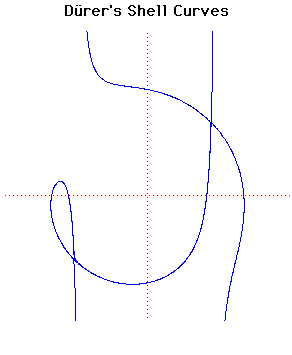# Curves

### Durer's Shell CurvesCartesian equation:
$(x^{2} + xy + ax - b^{2})^{2} = (b^{2} - x^{2})(x - y + a)^{2}$

### Description

These curves appear in Dürer's work Instruction in measurement with compasses and straight edge(1525).

Dürer calls the curve 'ein muschellini' which means a conchoid, but since it is not a true conchoid we have called it Dürer's shell curve (muschellini = conchoid = shell).

This curve arose from Dürer's work on perspective. He constructed the curve in the following way. He drew lines $QRP$ and $P'QR$ of length 16 units through $Q (q, 0)$ and $R (0, r)$ where $q + r = 13$. The locus of $P$ and $P'$ as $Q$ and $R$ move on the axes is the curve. Dürer only found one of the two branches of the curve.

The envelope of the line $P'QRP$ is a parabola and the curve is therefore a glissette of a point on a line segment sliding between a parabola and one of its tangents.

There are a number of interesting special cases:
In the above formula we have:

$b = 0$ : Curve becomes two coincident straight lines$x^{2} = 0$.

$a = 0$ : Curve becomes the line pair $x = b/√2, x = -b/√2$
together with the circle $x^{2} + y^{2} = b^{2}$.

$a = b/2$ : The curve has a cusp at $(-2a, a)$.

### Associated Curves

Definitions of the Associated curves